# Calculated columns change position?

I have this crosstable with a mix of measures and calculations. This is how I would like it to be presented (yellow marks calculations):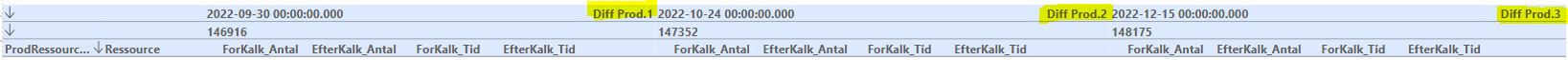This is designed to only be shown when you have selected an item number.

My problem is that when I have saved the dashboard and open it again or just choose another item number, then the calculated columns have shifted position to their default on the right.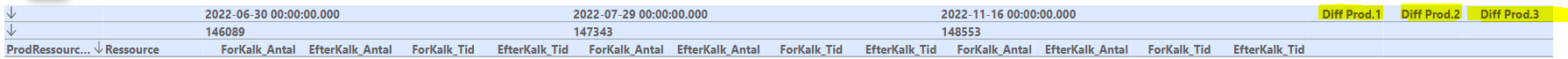Is there any way to lock the position?

• Can't you just make your calculation as a new measure (Nyt beregningsfelt) instead of a calculated column?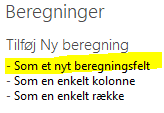Then it will appear next to your last measure for each item.

Or maybe I misunderstand your question?

• You are probably right, but then I think I need a little help to get the calculation right.

How would that look if I want the calculation to show the difference in % between "ForKalk Tid" and "EfterKalk Tid" ?

I can't seem to get it right...

• It depends on your measures.

if "ForKalk Antal" = m1, "EfterKalk Antal"  m2, "ForKalk Tid" = m3 and "EfterKalk Tid" = m4 I would guess your syntax should be something like: sum(0,0,m3)/sum(0,0,m4)*100

In my example below I have just made a test with 2 measures: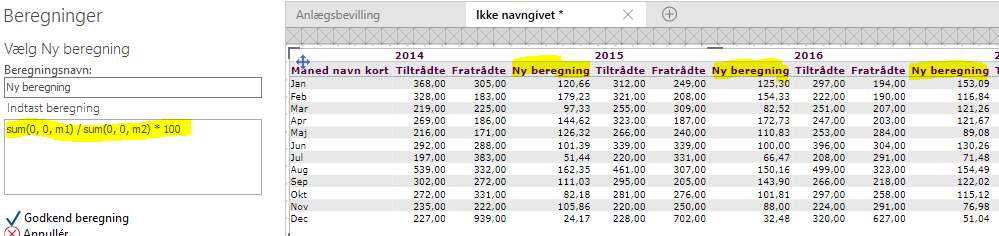If you are in doubt how to refer to a specific column or measure, you can use the advanced calculation. When you click on a column, you automatically get the syntax of the specific column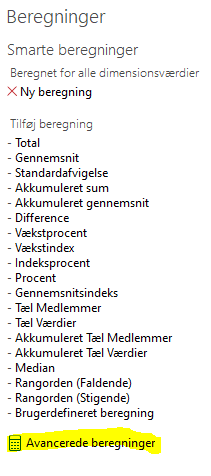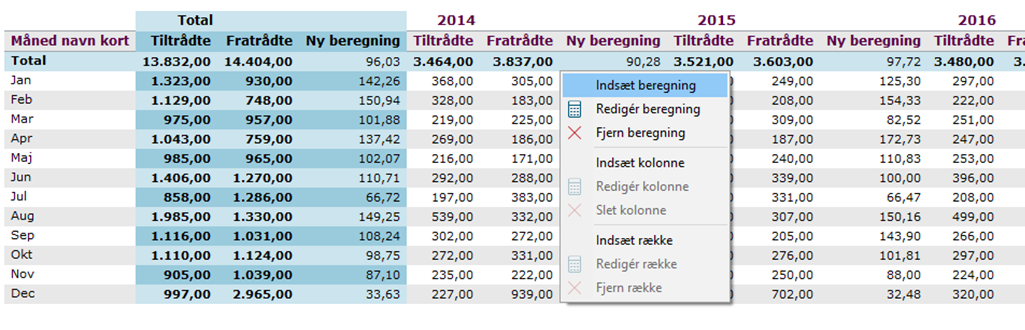But in this case it is probably not useful as it will give you the syntax of the actual row and dimension category e.g. sum(d-4,d-10,m3), which is not what you need.

The 0's in sum(0,0,m3) that I suggested, refer to the same row and the same dimension category as the calculated cell.

I hope it makes sence :-)

• Thank you Louise, that worked perfect... I had tried through advanced calculations, but as you suggest it referred to the exact column...

Now that you have been so helpful, maybe you can also help me with the last problem I have with this cross table.

Now I have these %-calculations. But as the example here shows, for some lines I get "Beregningsfejl", because the calculation divides with zero, which of course is not possible.

Is it possible to hide "Beregningsfejl" somehow?

• I don't know if you can make a visibility agent that hides "Beregningsfejl". But I always solves this problem with an if-sentence:

if sum(0,0,m4)=0 then 0 else sum(0,0,m3)/sum(0,0,m4)*100

And if you prefer that the cells is empty instead, you could say

if sum(0,0,m4)=0 then 999999 else sum(0,0,m3)/sum(0,0,m4)*100

And then make a visibility agent that hides all cells with 999999

• once again, thank you....

Now I can go on holiday ;-)